Courses

# RD Sharma Solutions - Chapter 2 - Powers (Ex-2.2) Part- 2 Class 8 Math Class 8 Notes | EduRev

## Class 8 : RD Sharma Solutions - Chapter 2 - Powers (Ex-2.2) Part- 2 Class 8 Math Class 8 Notes | EduRev

The document RD Sharma Solutions - Chapter 2 - Powers (Ex-2.2) Part- 2 Class 8 Math Class 8 Notes | EduRev is a part of the Class 8 Course RD Sharma Solutions for Class 8 Mathematics.
All you need of Class 8 at this link: Class 8

Question 8: By what number should (−5) −1 be multiplied so that the product is (−8) −1

Let the number be x.
According to the question,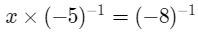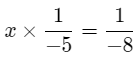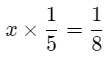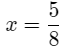Question 9: By what number should (15) −1 be multiplied so that the product may be equal to (−5) −1 ?

Let the required number be x.
Therefore,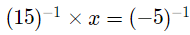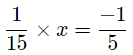x=−3
Hence, the required number is 3.

Question 10:

By what number should (−15)−1 be divided so that the quotient may be equal to (−5)−1?

Expressing in fractional form, we get:
(−15)−1 = −1/15,      ---> (a−1 = 1/a)
and
(−5)−1 = −1/5           ---> (a−1 = 1/a)
We have to find a number x such that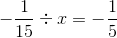Solving this equation, we get: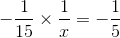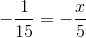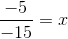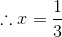Hence, (−15)−1 should be divided by 1/3 to obtain (−5)−1.

Question 11:

By what number should (5/3)−2  be multiplied so that the product may be (73)−1?

Expressing as a positive exponent, we have: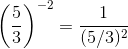---> (a−1 = 1/a)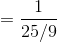---> ((a/b)n = (an)/(bn))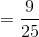and(7/3)−1 = 3/7.                ---> (a−1 = 1/a)
We have to find a number x such that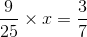Multiplying both sides by 25/9, we get: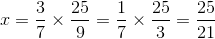Hence, (5/3)−2 should be multiplied by 25/21 to obtain (7/3)−1.

Question 12:

Find x, if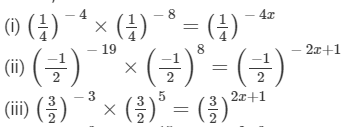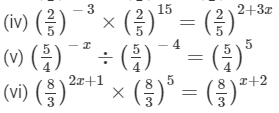(i) We have: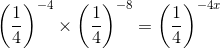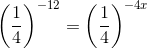(am×an = am+n)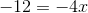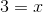x = 3
(ii) We have: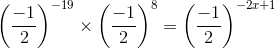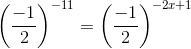(am×an = am+n)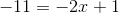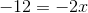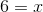x = 6
(iii) We have: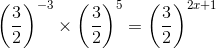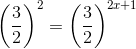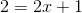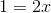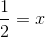x = 1/2
(iv) We have: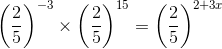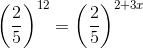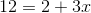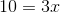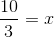x = 10/3
(v) We have: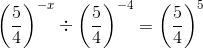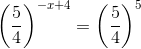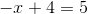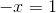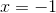(vi) We have: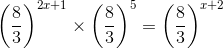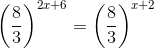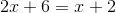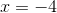Question 13: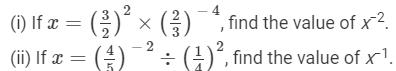(i) First, we have to find x.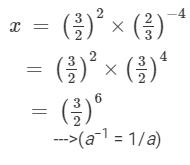x = 322×23-4  = Hence, x−2 is: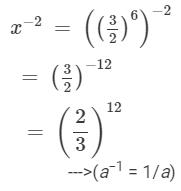(ii) First, we have to find x.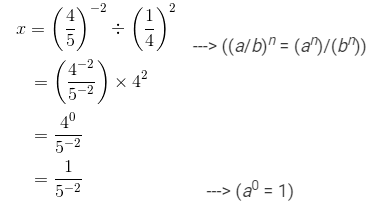Hence, the value of x−1 is: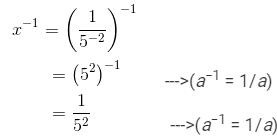Question 14: Find the value of x for which 52x ÷ 5−3 = 55.
We have: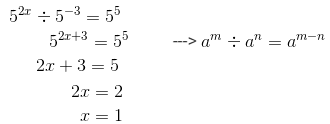hence, x is 1.

91 docs

,

,

,

,

,

,

,

,

,

,

,

,

,

,

,

,

,

,

,

,

,

;